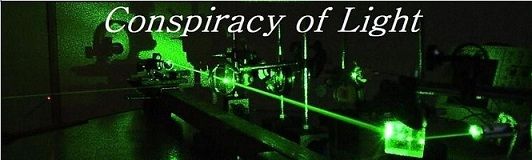The search for  new physics.

Theory and Experimental Tests:  The Nature of Space and Time.
The following papers discuss theories on the nature of space and time, and experiments that have been performed to determine whether the speed of light is constant in all directions as measured from Earth.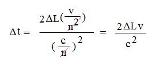In most interferometer experiments to date, do ether theory and relativity theory actually predict the same results? We explain why this is so, and develop a series of equations to apply to interferometer experiments to understand them in terms of ether theory.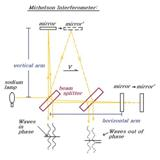Discusses the Michelson and Morley experiment, and how the results are consistent with both relativity, Lorentz Ether Theory (LET), and ether theories based on gravitational frame dragging.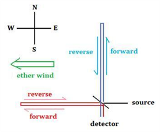A webapp that allows one to simulate the Michelson and Morley experiment and set a variety of conditions to determine what amount of fringe shift would be detected when the device is rotated from 0 to 90 degrees. Variables include Lorentz contraction, refractive index, relative speed of frame (ether wind), path length, and wavelength.  Uses equations developed in "The Premise for Comparing Ether Theory to Relativity Theory" above.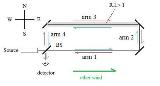Discusses the Hoek experiment with the propagation of light in a medium with a refractive index greater than 1, and how the results are consistent with both special relativity and Lorentz Ether Theory (LET).A webapp that allows one to simulate the Hoek experiment and set a variety of conditions to determine what amount of fringe shift would be detected when the device is rotated from 0 to 90 degrees. Variables include Lorentz contraction, refractive index, relative speed of frame (ether wind), path length, and wavelength.  Uses equations developed in "The Premise for Comparing Ether Theory to Relativity Theory" above.

6) The Michelson-Gale Experiment (1925)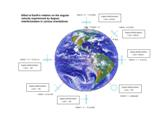Discusses the Michelson-Gale experiment and explains how it can be understood as a Sagnac Interferometer rotating at a latitude-dependent angular velocity.

7) Interactive Michelson-Gale Interferometer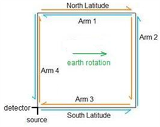A webapp that allows one to simulate the Michelson-Gale experiment. The original parameters are pre-loaded. The dimensions of the experiment, the latitude, the light wavelength etc. can be adjusted to see they effect the experimental outcome.

8) Interactive Sagnac Interferometer /Fibre-Optic Gyroscope (FOG)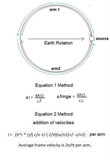A webapp that allows one to simulate the Sagnac effect. The original parameters of the Michelson-Gale experiment are pre-loaded. Various parameters can be adjusted to see how rotation, translation, and the movement of the earth affect the readings of the interferometer.

9) Gravitational Time Dilation - A Lorentzian Interpretation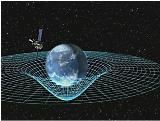An in depth discussion of how gravitational  time dilation can be explained by velocity effects, and using the reasoning of Lorentzian ether theory, a new model of space is derived which is curved in the medium of 3D space rather than in 4D space-time. The article also covers the Hafele and Keating experiment, and models for the time dilation of clocks at sea level (earths geoid).

10) Interactive Hafele and Keating Time Dilation ExperimentA webapp to simulate the around the world time dilation experiment using   clocks flown on planes westbound and eastbound. The time lost or gained on each clock it determined with respect to a clock that remains on the ground at the starting point. The simulator can vary parameters such as the altitudes the clocks are flown at, the latitude of the path over the surface of the earth, and the total trip time for each flight. The individual terms (velocity, Sagnac and height) are calculated and then shown separately and as a net time gain/loss by each clock.

11)  Interactive Gravitational Potential and Coordinate Time Increment on Earth's Oblate Geoid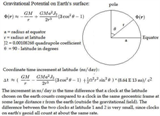A webapp to simulate the textbook calculation of gravitational potential on the earth's surface, and the coordinate time increment of clocks at different latitudes at sea level.

12)  Interactive Gravitational Potential and Coordinate Time Increment on Earth's Oblate GeoidA more advanced webapp simulation also comparing time dilation effects on moving clocks at velocities and heights over the earth's surface, similar to the Hafele and Keating experiment.Discusses an alternative explanation for relativistic time dilation.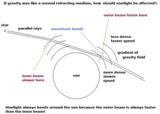Discusses Einstein's 1911 paper "On  the  Influence  of Gravitation  on  the Propagation of Light.", proposes an alternate theory of gravitational refraction by the medium of space, and examines the results of the Pound and Rebka Experiment.

15) The Use of Moved Clocks: How this Challenges Special Relativity.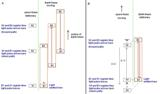Einstein time synchronization excludes the possibility that the speed of light is not constant in both directions. We show that if clocks and lengths are synchronized, at least one moving frame must have a one-way velocity of light differing from C.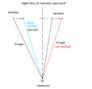Discusses Einstein's ideas regarding the Relativistic Transverse Doppler Effect. and how the non-classical part of this effect can be alternatively explained as being due to a Lorentz contraction of the moving source as suggested by Herbert Ives.Transverse Doppler Effect. Discute des idées d'Einstein concernant l'effet Doppler transverse relativiste. et comment la partie non-classique de cet effet peut être alternativement expliquée comme étant due à une contraction de Lorentz de la source mobile comme suggéré par Herbert Ives. La version en langue française est ici:  Effet Doppler Transverse.

17) Some Notes on Understanding the Schrodinger Wave Equation.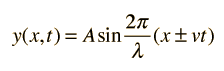Our attempt to work through the math involved in Schrodinger's wave equation, from the perspective of understanding how Schrodinger used de Broglie's idea of an electron as a wave to model the hydrogen atom.

18) The Continuing Relevance of Lorentz Ether Theory in the Age of RelativityWith the advent of relativity the Lorentz ether theory has been relegated to not much more than a historical footnote.  What is less well known is that virtually all optical experiments to date to test the validity of special relativity cannot distinguish between the predicted outcomes of the two theories.  We review the historical development of the Lorentz theory as it evolved to address the results of key optical observations of the 19th century.  We then examine how modern optical experiments attempting to detect the relative inertial motion of an observer with respect to a preferred reference frame for light remain consistent with Lorentz’s predictions. A french version of this article is available here.Presentation at the NPA-18 conference, July 6th, 2011 of the paper "The Continuing Relevance of Lorentz Ether Theory in the Age of Relativity".A series of thought experiments showing how the Sagnac effect contravenes in principle the conceptsof the relativity of time and motion. Une série d'expériences de pensée montrant comment l'effet Sagnac contrevient en principe au concept de la relativité du temps et du mouvement. Pour une version en langue française, cliquez ici: L'expérience Sagnac: est-ce en contradiction avec la Relativité?Hafele and Keating performed a round the world time dilation experiment using planes in 1971. Although the experiment is usually applauded as a great experimental verification of relativity, on deeper examination it is found to require the adoption of a concept of absolute reference frames for inertial and rotational motion that would appear to put relativity theory in contradiction wioth its own underlying tenets.

22) The Kennedy-Thorndike Experiment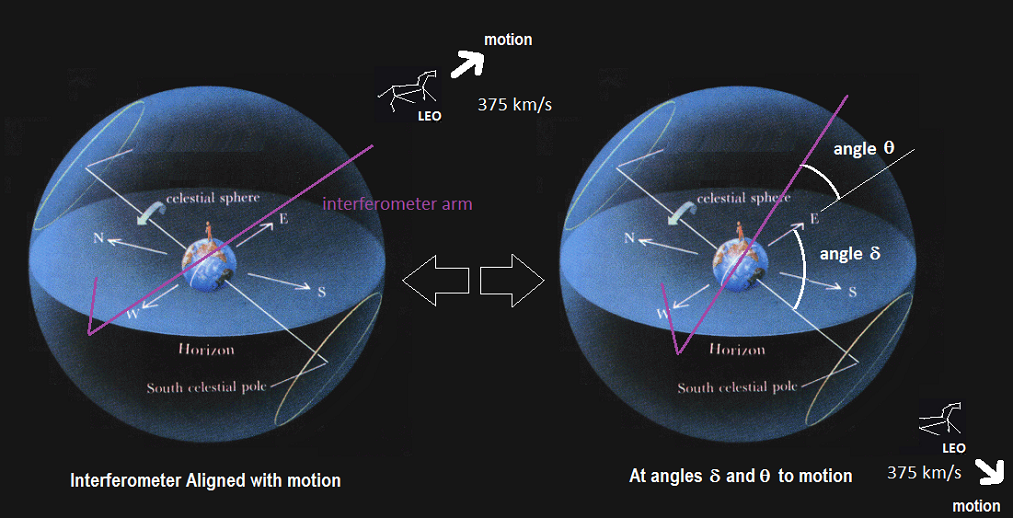A discussion of the Kennedy - Thorndike Experiment and a simulation with examples to show how the experiment is consistent with the predictions of Lorentz ether theory.The Lorentz force is usually described abstractly without reference to the physical mechanism by which the force acts in space. We show Maxwell's physical model based on magnetic lines of force as vortex tubes in a medium.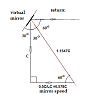We attempt to show why terrestrial aberration does not occur in a Michelson interferometer. The light beams are shown to arrive at the interferometer detector perfectly parallel despite any velocity, due to a physical Lorentz contraction of the apparatus and the beamsplitter.We explain why a physical Lorentz contraction of matter with velocity would be consistent with the wave nature of matter discovered by the Nobel laureates de Broglie and Schrodinger.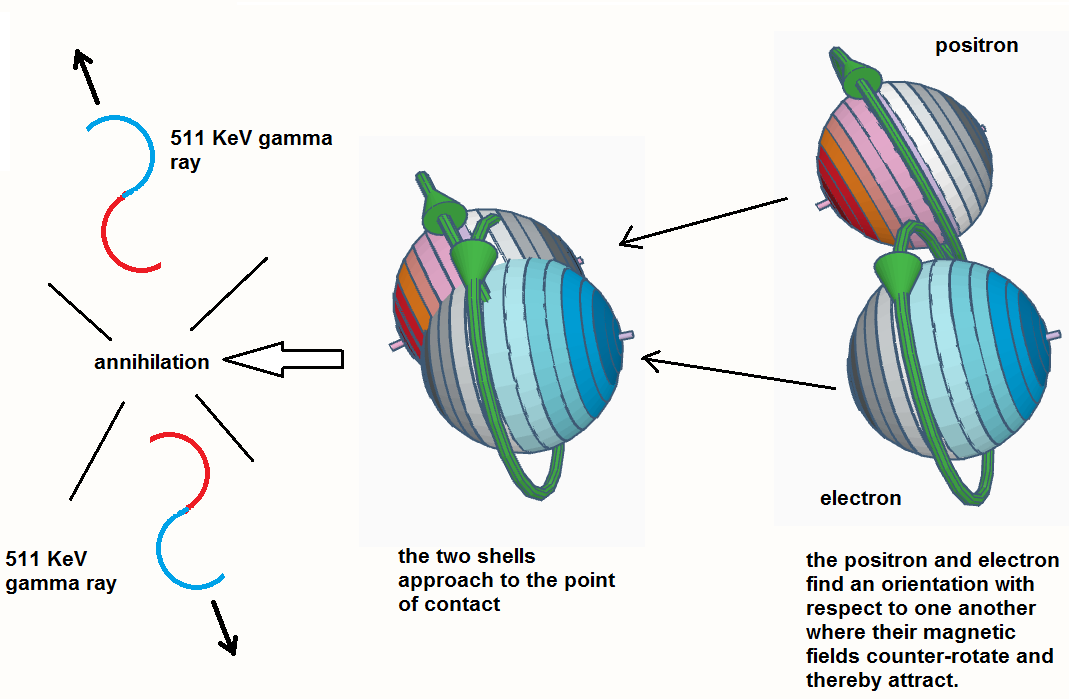We explain Schrodinger's physical model of the electron and the atom as he appears to have conceived of it between 1926 to 1933 when he received the Nobel prize.  We also dicuss the shortcomings of this model and some possible ways to overcome them.We explain why a physical Lorentz contraction of matter with velocity would be consistent with the wave nature of matter discovered by the Nobel laureate Schrodinger, using his spherical wave model of the electron.

28) TBA